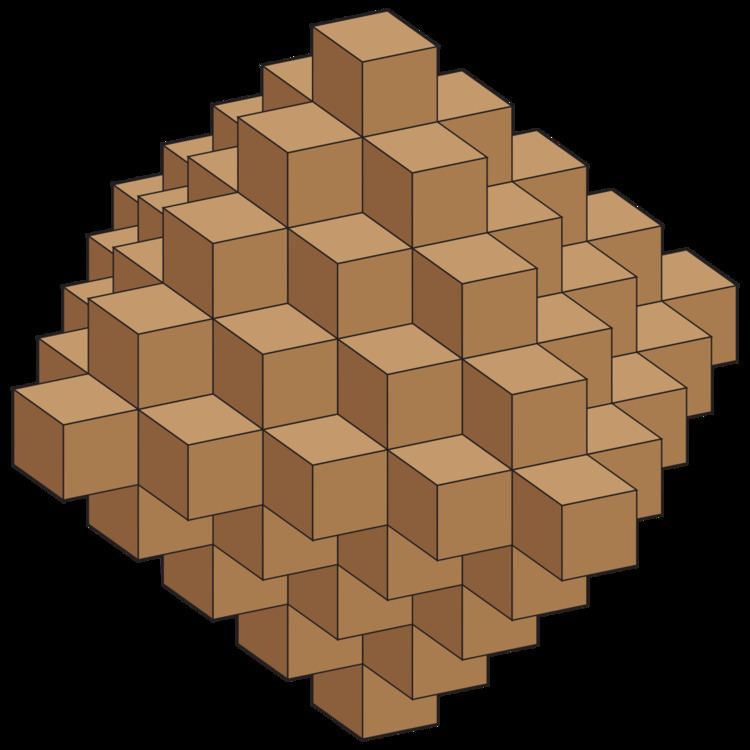# Centered octahedral number

Updated on
Covid-19A centered octahedral number or Haüy octahedral number is a figurate number that counts the number of points of a three-dimensional integer lattice that lie inside an octahedron centered at the origin. The same numbers are special cases of the Delannoy numbers, which count certain two-dimensional lattice paths. The Haüy octahedral numbers are named after René Just Haüy.

## History

The name "Haüy octahedral number" comes from the work of René Just Haüy, a French mineralogist active in the late 18th and early 19th centuries. His "Haüy construction" approximates an octahedron as a polycube, formed by accreting concentric layers of cubes onto a central cube. The centered octahedral numbers count the number of cubes used by this construction. Haüy proposed this construction, and several related constructions of other polyhedra, as a model for the structure of crystalline minerals.

## Formula

The number of three-dimensional lattice points within n steps of the origin is given by the formula

( 2 n + 1 ) × ( 2 n 2 + 2 n + 3 ) 3

The first few of these numbers (for n = 0, 1, 2, ...) are

1, 7, 25, 63, 129, 231, 377, 575, 833, 1159, …

The generating function of the centered octahedral numbers is

( 1 + x ) 3 ( 1 x ) 4 .

The centered octahedral numbers obey the recurrence relation

C ( n ) = C ( n 1 ) + 4 n 2 + 2.

They may also be computed as the sums of pairs of consecutive octahedral numbers.

## Alternative interpretations

The octahedron in the three-dimensional integer lattice, whose number of lattice points is counted by the centered octahedral number, is a metric ball for three-dimensional taxicab geometry, a geometry in which distance is measured by the sum of the coordinatewise distances rather than by Euclidean distance. For this reason, Luther & Mertens (2011) call the centered octahedral numbers "the volume of the crystal ball".

The same numbers can be viewed as figurate numbers in a different way, as the centered figurate numbers generated by a pentagonal pyramid. That is, if one forms a sequence of concentric shells in three dimensions, where the first shell consists of a single point, the second shell consists of the six vertices of a pentagonal pyramid, and each successive shell forms a larger pentagonal pyramid with a triangular number of points on each triangular face and a pentagonal number of points on the pentagonal face, then the total number of points in this configuration is a centered octahedral number.

The centered octahedral numbers are also the Delannoy numbers of the form D(3,n). As for Delannoy numbers more generally, these numbers count the number of paths from the southwest corner of a 3 × n grid to the northeast corner, using steps that go one unit east, north, or northeast.

Similar Topics
Rang (2014 film)
Josh Abramson
Vincent Mason
Topics